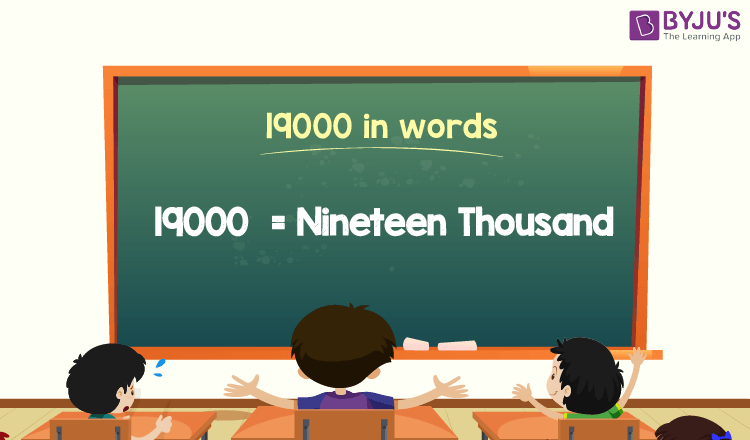# 19000 in Words

19000 in words is Nineteen Thousand. For example, if you spent 19000 this month, then you can write, “I have spent nineteen thousand this month”. To write the number name of 19000, the place value chart is beneficial.

 19000 in Words: Nineteen Thousand

In this article, let us discuss how to write the number 19000 in words, i.e., the spelling of 19000, and look at many solved examples in detail.

## How to Write 19000 in Words?The number 19000 in words is written using the place value of a number. Thus, to write the 19000 in words, identify the place value of each digit of 19000.

For a number 19000,

The digit in one’s place is 0.

The digit in ten’s place is 0.

The digit in hundred’s place is 0.

The digit in thousand’s place is 19.

Thus, the place value chart to represent the number 19000 is:

 Thousands Hundreds Tens Ones 19 0 0 0

Therefore, the number 19000 in words is Nineteen Thousand.

### Examples

Example 1:

Express the value of 15000 + 4000 in words.

Solution:

Given expression: 15000 + 4000

⇒ 15000 + 4000 = 19000

So, the value of 15000 + 4000 is 19000.

Hence, 19000 in words is nineteen thousand.

Example 2:

Find the value of nineteen thousand plus two thousand. Express the value in words.

Solution:

Nineteen thousand = 19000

Two thousand = 2000

Nineteen thousand plus two thousand = 19000 + 2000 = 21000

Hence, the value of nineteen thousand plus two thousand is twenty-one thousand in words.

Stay tuned to BYJU’S – The Learning App, and download the app today to learn all Maths concepts.

## Frequently Asked Questions on 19000 in Words

Q1

### Write 19000 in words.

19000 in words is nineteen thousand.

Q2

### Find the value of 20000 – 1000, and express the answer in words.

Simplifying 20000 – 1000, we get 19000. Hence, 19000 in words is nineteen thousand.

Q3

### How to write nineteen thousand in numbers?

Nineteen thousand in numbers is 19000.